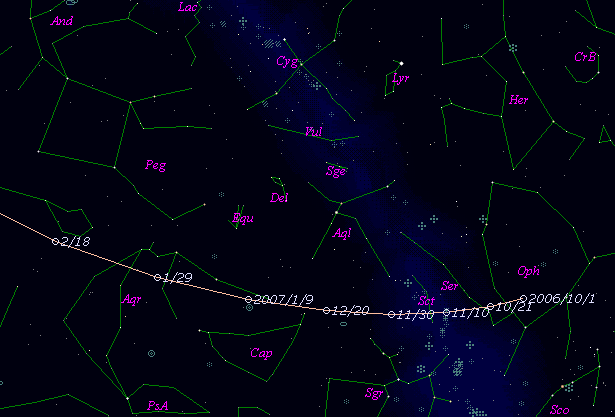# \$B%Z%H%j%e!

185P/Petriew (2007)###\$B%W%m%U%#!<%k(B

 \$BId9f(B 185P/2007 A3 \$B8!=PF|(B 2007\$BG/(B1\$B7n(B11\$BF|(B \$B8!=P8wEY(B 16.1\$BEy(B \$B8!=P F. Fratev (E. Mihaylova), A. Kirchev (Sofia, Bulgaria)

###\$B###\$B50F;MWAG(B

```                    Epoch = 2007 Mar.  1.0 TT
T = 2007 Feb. 24.5806 TT         Peri. = 181.9215
e = 0.698129                     Node  = 214.1025  2000.0
q = 0.937602 AU                  Incl. =  13.9747
a =  3.105970 AU    n = 0.1800563    P =   5.47 years
```

###\$B@1?^(B###\$B8wEYJQ2=(B

```        m1 = 11.3 + 5 log\$B&\$(B + 30.0 log r(t - 10)
```##### \$B50F;MWAG\$O(B Dr. Brian G. Marsden \$B\$N7W;;\$K\$h\$k\$b\$N\$G!"(B IAUC 8795 \$B\$K7G:\\$5\$l\$?\$b\$N\$G\$9!#(B \$B@1?^\$O(B StellaNavigator Ver.2.0 for Windows (\$B%"%9%H%m%"!<%D(B \$BJTCx(B / \$B%"%9%-!<=PHG6I4)(B) \$B\$G:n@.\$7\$?\$b\$N\$G\$9!#(B \$B8wEY%0%i%U\$O(BComet for Windows\$B\$G:n@.\$7\$?\$b\$N\$G\$9!#(B